# Resolving forcesPage 2

#### WATCH ALL SLIDES

Slide 14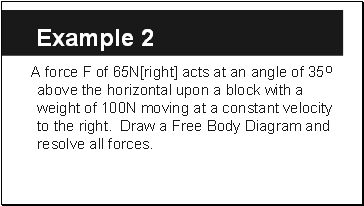Example 2

A force F of 65N[right] acts at an angle of 35O above the horizontal upon a block with a weight of 100N moving at a constant velocity to the right. Draw a Free Body Diagram and resolve all forces.

Slide 15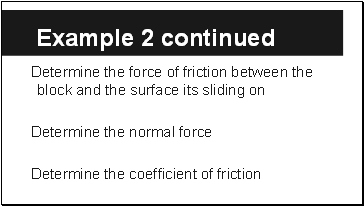Example 2 continued

Determine the force of friction between the block and the surface its sliding on

Determine the normal force

Determine the coefficient of friction

Slide 16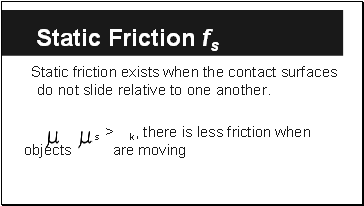## Static Friction fs

Static friction exists when the contact surfaces do not slide relative to one another.

s > k, there is less friction when objects are moving

Slide 17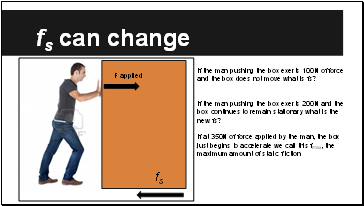## fs can change

If the man pushing the box exerts 100N of force and the box does not move what is fs?

If the man pushing the box exerts 200N and the box continues to remain stationary what is the new fs?

If at 350N of force applied by the man, the box just begins to accelerate we call this fmax, the maximum amount of static friction

F applied

fs

Slide 18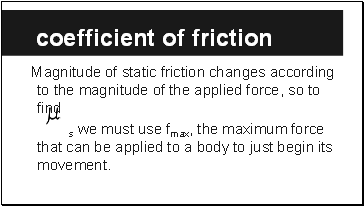## Coefficient of friction

Magnitude of static friction changes according to the magnitude of the applied force, so to find

s we must use fmax, the maximum force that can be applied to a body to just begin its movement.

Slide 19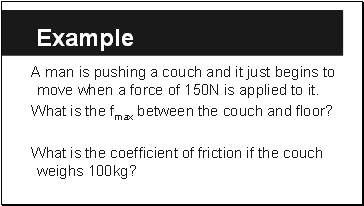Example

A man is pushing a couch and it just begins to move when a force of 150N is applied to it.

What is the fmax between the couch and floor?

What is the coefficient of friction if the couch weighs 100kg?

Slide 20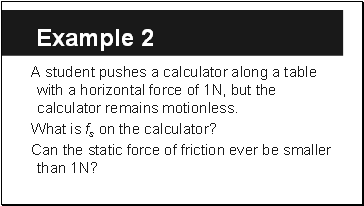Example 2

A student pushes a calculator along a table with a horizontal force of 1N, but the calculator remains motionless.

What is fs on the calculator?

Can the static force of friction ever be smaller than 1N?

Slide 21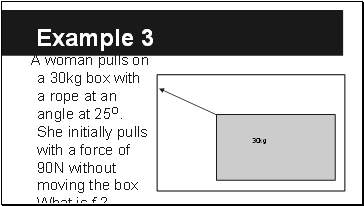Example 3

A woman pulls on a 30kg box with a rope at an angle at 25O. She initially pulls with a force of 90N without moving the box What is fs?

30kg

Go to page:
1  2